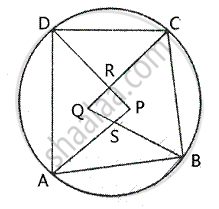Share

The Quadrilateral Formed by Angle Bisectors of a Cyclic Quadrilateral is Also Cyclic. Prove It. - ICSE Class 10 - Mathematics

Question

The quadrilateral formed by angle bisectors of a cyclic quadrilateral is also cyclic. Prove it.

SolutionGiven – ABCD is a cyclic quadrilateral and PQRS is a
Quadrilateral formed by the angle Bisectors of angle ∠A, ∠B , ∠C and ∠D
To prove – PQRS is a cyclic quadrilateral. Proof – In APD ∠PAD + ∠ADP + ∠APD = 180°    .…. (1)
Similarly, IN ∆BQC,
∠QBC + ∠BCQ + ∠BQC = 180°        …………(2)

Adding (1) and (2) .we get
∠PAD + ∠ADP + ∠APD + ∠QBC + ∠BCQ + ∠BQC = 180° +180°
∠PAD + ∠ADP + ∠QBC + ∠BCQ + ∠APD + ∠BQC = 360°
But ∠PAD + ∠ADP + ∠QBC + ∠BCQ =1/2 [∠A + B + ∠C + ∠D]

= 1/2 xx 360° = 180°
∴ ∠APD + ∠BQC = 360° -180° = 180°         [from (3)]
But these are the sum of opposite angles of quadrilateral PRQS.
∴ Quad. PRQS is a cyclic quadrilateral.

Is there an error in this question or solution?
Solution The Quadrilateral Formed by Angle Bisectors of a Cyclic Quadrilateral is Also Cyclic. Prove It. Concept: Cyclic Properties.
S